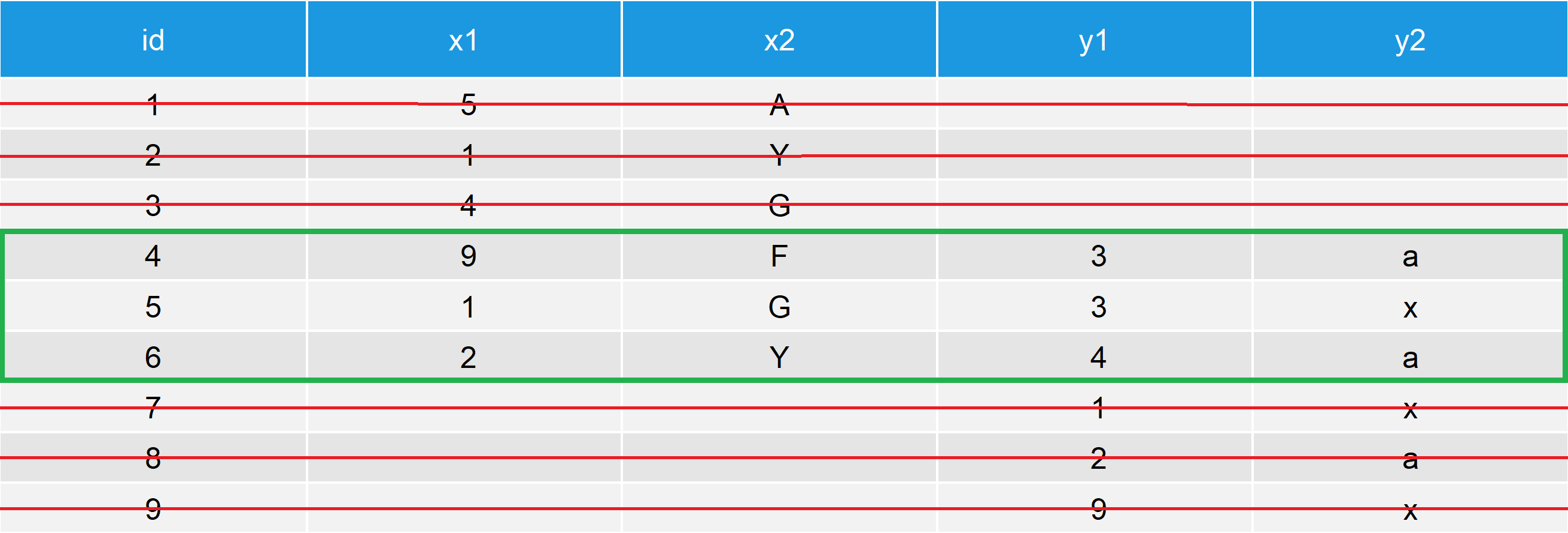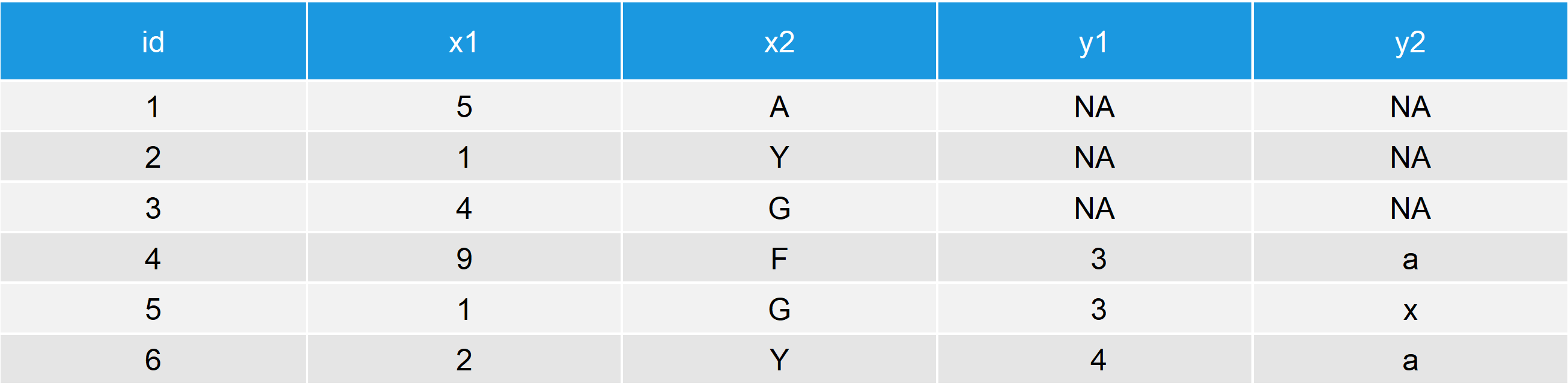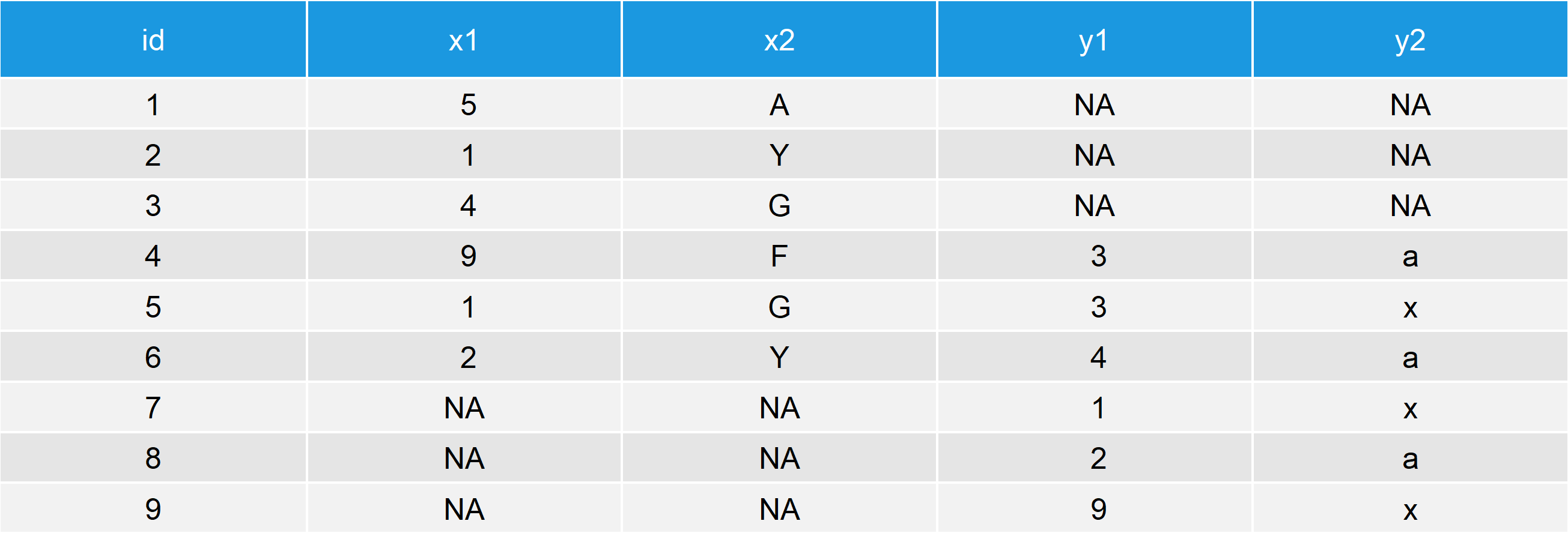# Merge Data Frames by Column Names in R (3 Examples)

In this R post you’ll learn how to merge data frames by column names.

The tutorial consists of three examples for the merging of different data sets. More precisely, the article consists of the following contents:

You’re here for the answer, so let’s get straight to the examples…

## Example 1: Basic Application of merge Function in R

First, we need to create some data frames that we can use in the example later on. Consider the following R code:

```data1 <- data.frame(id = 1:6,                                  # Create first example data frame
x1 = c(5, 1, 4, 9, 1, 2),
x2 = c("A", "Y", "G", "F", "G", "Y"))

data2 <- data.frame(id = 4:9,                                  # Create second example data frame
y1 = c(3, 3, 4, 1, 2, 9),
y2 = c("a", "x", "a", "x", "a", "x"))```

Each of our two example data frames contains three columns. Both data frames have an id column (e.g. an identification number for a household).

We can now use the merge() R function to combine our two data frames by the id column as follows:

`merge(data1, data2, by = "id")                                 # Merge data frames by columns names`Table 1: Basic Merging of Two Data Frames.

Table 1 illustrates the merging process of the two data frames. As you can see based on the table, the two data frames have the id values 4, 5, and 6 in common. The ids 1, 2, 3, 7, 8, and 9 were therefore not kept in the joined data frame. In statistical research literature, this type of merging is often called inner join.

Do you want to keep the rows of your data frames even when they are not matched? Then keep reading, this is what I’m going to show you next…

## Example 2: Keep Data of Unmatched Rows

The merge function provides the options all.x and all.y. These two options can be used to retain certain rows of your input data tables, even when no match is found for the merging.

With the following R codes, we can keep all rows of our first input data frame (i.e. data1)…

`merge(data1, data2, by = "id", all.x = TRUE)                   # Keep all rows of x-data`Table 2: Keep All Rows of X-Data.

…of our second input data frame (i.e. data2)…

`merge(data1, data2, by = "id", all.y = TRUE)                   # Keep all rows of y-data`Table 3: Keep All Rows of Y-Data.

…or we can even keep all rows of both data files:

`merge(data1, data2, by = "id", all.x = TRUE, all.y = TRUE)     # Keep all rows of both data frames`Table 4: Keep All Rows of Both Data Frames.

Note: Observations for which no match is found are set to NA in the corresponding column(s).

The three joining types that I have shown in Example 2 are often named as left join, right join, and full join. You can learn more about the different types of SQL joins here.

## Example 3: Merge Multiple Data Frames

So far, we have only merged two data tables. In reality, however, we will often have to merge multiple data frames to a single data matrix.

Fortunately, in the R programming language this can be achieved easily with a step-by-step approach. Let’s see how this looks in practice.

First, we need to create a third example data set:

```data3 <- data.frame(id = 5:6,                                  # Create third example data frame
z1 = c(3, 2),
z2 = c("K", "b"))```

Now, we can simply merge our three data frames one after each other. First, I’m going to create a combined data set of data1 and data2…

`data12 <- merge(data1, data2, by = "id")                       # Merge data 1 & 2 and store`

…and then I’m going to join the third example data set to my previously merged data set, leading to a single data set containing the rows with all shared ids of our three data matrices without losing data:

`merge(data12, data3, by = "id")                                # Merge multiple data frames`Table 5: Three Merged Data Frames.

In the previous R syntax, I applied an inner join, but of cause you could also use a right, left, or full join in this step-by-step approach.

Also note that there is a very smooth way to merge multiple data frames simultaneously by combining these data frames in a list. You can learn more about this approach in this tutorial.

## Video, Further Resources & Summary

In case you need further explanations for the application of the merge function in R, you could have a look at the following video of my YouTube channel. In the video, I’m explaining the examples of this tutorial in a live programming session in RStudio.

Please accept YouTube cookies to play this video. By accepting you will be accessing content from YouTube, a service provided by an external third party.If you accept this notice, your choice will be saved and the page will refresh.

Please also note that the merge command is only one of several functions, which allow for combining several data sources. Below, you can find a list of tutorials that explain the merge function in more complex settings as well as the usage of other merging functions such as rbind, cbind, or the different join functions of the dplyr package. Check out the list below to see if one of these tutorials is fitting your data situation more adequately:

Finally, you could also have a look at some of the other R tutorials of my website. You can find good overviews here:

In summary: This tutorial explained how to merge 2 or 3 data frames by a column vector in the R programming language. The tutorial has explained different types of joins such as inner joins and cross joins. Please let me know in case you have any further questions.

Subscribe to the Statistics Globe Newsletter

•Randy Mason
February 1, 2022 4:57 pm

Thank you for your fb posts. I find them helpful and allows me to practice.

•February 7, 2022 10:27 am